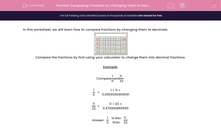# Comparing Fractions by Changing Them to Decimals

In this worksheet, students test the comparison of fractions by changing them to decimals.Key stage:  KS 2

Curriculum topic:   Maths and Numerical Reasoning

Curriculum subtopic:   Decimals

Difficulty level:#### Worksheet Overview

In this worksheet, we will learn how to compare fractions by changing them to decimals.Compare the fractions by first using your calculator to change them into decimal fractions.

Example:

Compare
 1 11
and
 11 23

 1 11
=
1 ÷ 11 = 0.0909090909091

 11 23
=
11 ÷ 23 = 0.478260869565

 1 11
is less than
 11 23

### What is EdPlace?

We're your National Curriculum aligned online education content provider helping each child succeed in English, maths and science from year 1 to GCSE. With an EdPlace account you’ll be able to track and measure progress, helping each child achieve their best. We build confidence and attainment by personalising each child’s learning at a level that suits them.

Get started